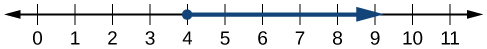## Writing and Manipulating Inequalities

### Learning Outcomes

• Use interval notation to express inequalities.
• Use properties of inequalities.

Indicating the solution to an inequality such as $x\ge 4$ can be achieved in several ways.

We can use a number line as shown below. The blue ray begins at $x=4$ and, as indicated by the arrowhead, continues to infinity, which illustrates that the solution set includes all real numbers greater than or equal to 4.We can use set-builder notation: $\{x|x\ge 4\}$, which translates to “all real numbers x such that x is greater than or equal to 4.” Notice that braces are used to indicate a set.

The third method is interval notation, where solution sets are indicated with parentheses or brackets. The solutions to $x\ge 4$ are represented as $\left[4,\infty \right)$. This is perhaps the most useful method as it applies to concepts studied later in this course and to other higher-level math courses.

The main concept to remember is that parentheses represent solutions greater or less than the number, and brackets represent solutions that are greater than or equal to or less than or equal to the number. Use parentheses to represent infinity or negative infinity, since positive and negative infinity are not numbers in the usual sense of the word and, therefore, cannot be “equaled.” A few examples of an interval, or a set of numbers in which a solution falls, are $\left[-2,6\right)$, or all numbers between $-2$ and $6$, including $-2$, but not including $6$; $\left(-1,0\right)$, all real numbers between, but not including $-1$ and $0$; and $\left(-\infty ,1\right]$, all real numbers less than and including $1$. The table below outlines the possibilities.

Set Indicated Set-Builder Notation Interval Notation
All real numbers between a and b, but not including a or b $\{x|a<x<b\}$ $\left(a,b\right)$
All real numbers greater than a, but not including a $\{x|x>a\}$ $\left(a,\infty \right)$
All real numbers less than b, but not including b $\{x|x<b\}$ $\left(-\infty ,b\right)$
All real numbers greater than a, including a $\{x|x\ge a\}$ $\left[a,\infty \right)$
All real numbers less than b, including b $\{x|x\le b\}$ $\left(-\infty ,b\right]$
All real numbers between a and b, including a $\{x|a\le x<b\}$ $\left[a,b\right)$
All real numbers between a and b, including b $\{x|a<x\le b\}$ $\left(a,b\right]$
All real numbers between a and b, including a and b $\{x|a\le x\le b\}$ $\left[a,b\right]$
All real numbers less than a or greater than b $\{x|x<a\text{ and }x>b\}$ $\left(-\infty ,a\right)\cup \left(b,\infty \right)$
All real numbers $\{x|x\text{ is all real numbers}\}$ $\left(-\infty ,\infty \right)$

### Example: Using Interval Notation to Express All Real Numbers Greater Than or Equal to a

Use interval notation to indicate all real numbers greater than or equal to $-2$.

### Try It

Use interval notation to indicate all real numbers between and including $-3$ and $5$.

### Example: Using Interval Notation to Express All Real Numbers Less Than or Equal to a or Greater Than or Equal to b

Write the interval expressing all real numbers less than or equal to $-1$ or greater than or equal to $1$.

### Try It

Express all real numbers less than $-2$ or greater than or equal to 3 in interval notation.

## Using the Properties of Inequalities

When we work with inequalities, we can usually treat them similarly to but not exactly as we treat equations. We can use the addition property and the multiplication property to help us solve them. The one exception is when we multiply or divide by a negative number, we must reverse the inequality symbol.

### A General Note: Properties of Inequalities

$\begin{array}{ll}\text{Addition Property}\hfill& \text{If }a< b,\text{ then }a+c< b+c.\hfill \\ \hfill & \hfill \\ \text{Multiplication Property}\hfill & \text{If }a< b\text{ and }c> 0,\text{ then }ac< bc.\hfill \\ \hfill & \text{If }a< b\text{ and }c< 0,\text{ then }ac> bc.\hfill \end{array}$

These properties also apply to $a\le b$, $a>b$, and $a\ge b$.

### Example: Demonstrating the Addition Property

Illustrate the addition property for inequalities by solving each of the following:

1. $x - 15<4$
2. $6\ge x - 1$
3. $x+7>9$

### Try It

Solve $3x - 2<1$.

### Example: Demonstrating the Multiplication Property

Illustrate the multiplication property for inequalities by solving each of the following:

1. $3x<6$
2. $-2x - 1\ge 5$
3. $5-x>10$

### Try It

Solve $4x+7\ge 2x - 3$.

## Solving Inequalities in One Variable Algebraically

As the examples have shown, we can perform the same operations on both sides of an inequality, just as we do with equations; we combine like terms and perform operations. To solve, we isolate the variable.

### Example: Solving an Inequality Algebraically

Solve the inequality: $13 - 7x\ge 10x - 4$.

### Try It

Solve the inequality and write the answer using interval notation: $-x+4<\frac{1}{2}x+1$.

### Example: Solving an Inequality with Fractions

Solve the following inequality and write the answer in interval notation: $-\frac{3}{4}x\ge -\frac{5}{8}+\frac{2}{3}x$.

### Try It

Solve the inequality and write the answer in interval notation: $-\frac{5}{6}x\le \frac{3}{4}+\frac{8}{3}x$.

## Contribute!

Did you have an idea for improving this content? We’d love your input.